# Motion Speed Velocity Acceleration What Is Motion Motion

• Slides: 26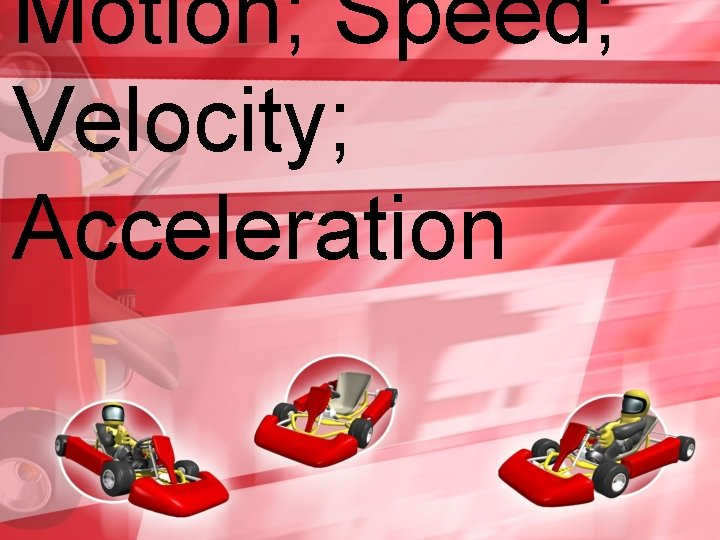Motion; Speed; Velocity; AccelerationWhat Is Motion? Motion is when an object changes place or position. To properly describe motion, you need to use the following: • • Start and end position? Movement relative to what? How far did it go? In what direction did it go?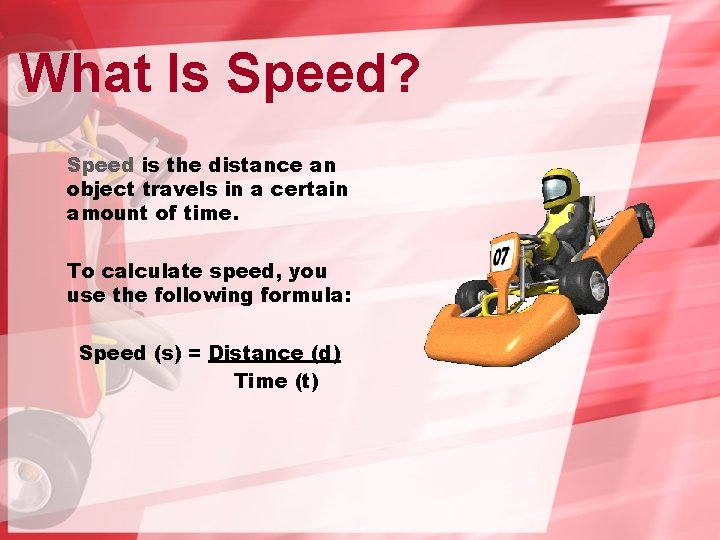What Is Speed? Speed is the distance an object travels in a certain amount of time. To calculate speed, you use the following formula: Speed (s) = Distance (d) Time (t)Speed Math Problem Suppose you ran 2 km in 10 min. What is your speed? S = 2 km = 0. 2 km/min 10 minAnother Problem What is the speed of a car traveling 144 km in 90 minutes per hour? ________ km/h In miles per hour? _____ mphSolution Km/hour: How many hours are in 90 minutes? There are 60 minutes in 1 hour; therefore 90 minutes equals 1. 5 hours 144 km = 96 km/h 1. 5 hours Miles per hour: How many km are in a mile? 1 km = 0. 621 miles; therefore 144 km = 89. 424 miles = 59. 616 mpg or 59. 6 mpg 1. 5 hours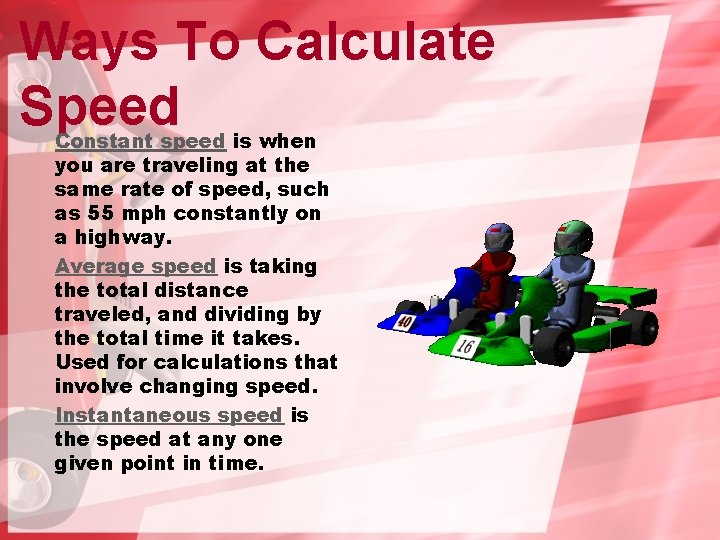Ways To Calculate Speed Constant speed is when you are traveling at the same rate of speed, such as 55 mph constantly on a highway. Average speed is taking the total distance traveled, and dividing by the total time it takes. Used for calculations that involve changing speed. Instantaneous speed is the speed at any one given point in time.Average Speed What is the AVERAGE speed of the bass boat depicted in the graph?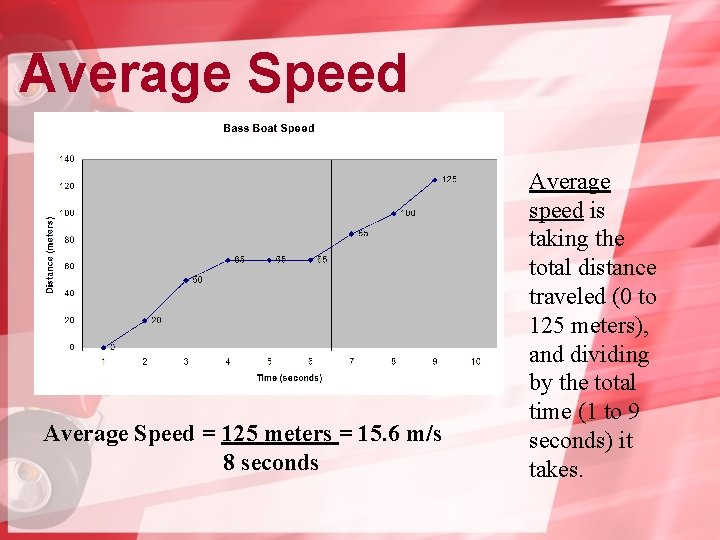Average Speed = 125 meters = 15. 6 m/s 8 seconds Average speed is taking the total distance traveled (0 to 125 meters), and dividing by the total time (1 to 9 seconds) it takes.Instantaneous Speed What is the instantaneous speed of the bass boat at t=7 seconds?Instantaneous Speed = 85 meters = 12. 1 m/s 7 seconds Instantaneous speed is speed at any given point in time. At 7 seconds, the distance is 85 meters; therefore the IS isSpeed Graphs • In what time period is the bass boat speeding up? • In what time period is the bass boat slowing down? • When is the speed NOT changing?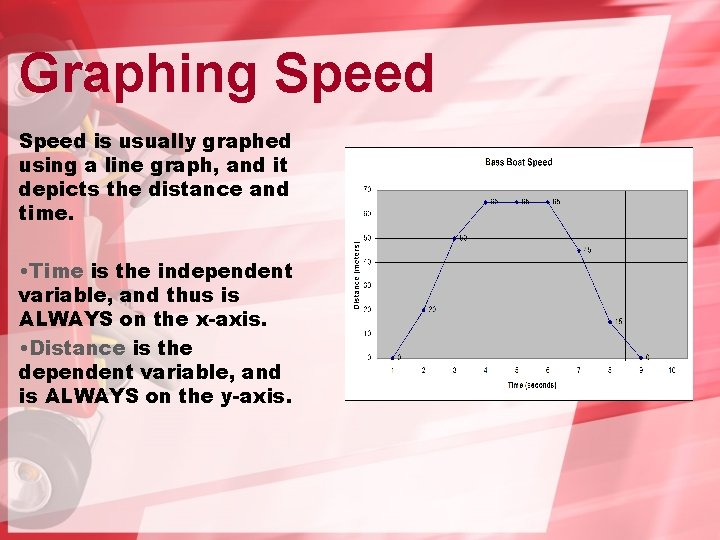Graphing Speed is usually graphed using a line graph, and it depicts the distance and time. • Time is the independent variable, and thus is ALWAYS on the x-axis. • Distance is the dependent variable, and is ALWAYS on the y-axis.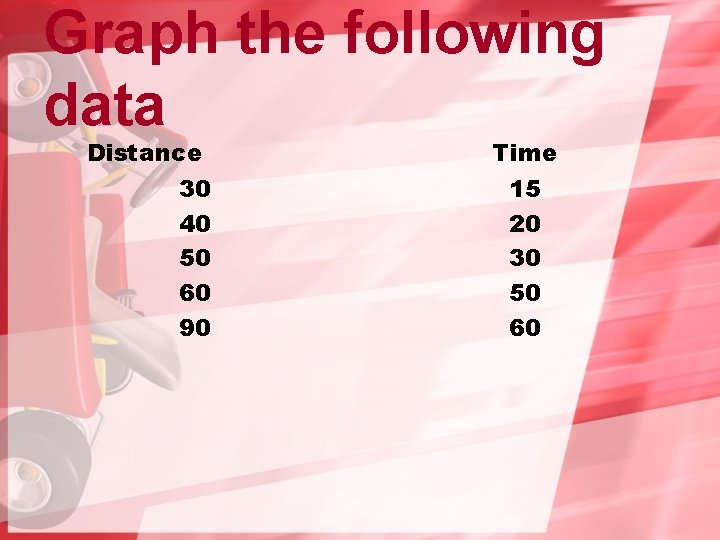Graph the following data Distance 30 40 50 60 90 Time 15 20 30 50 60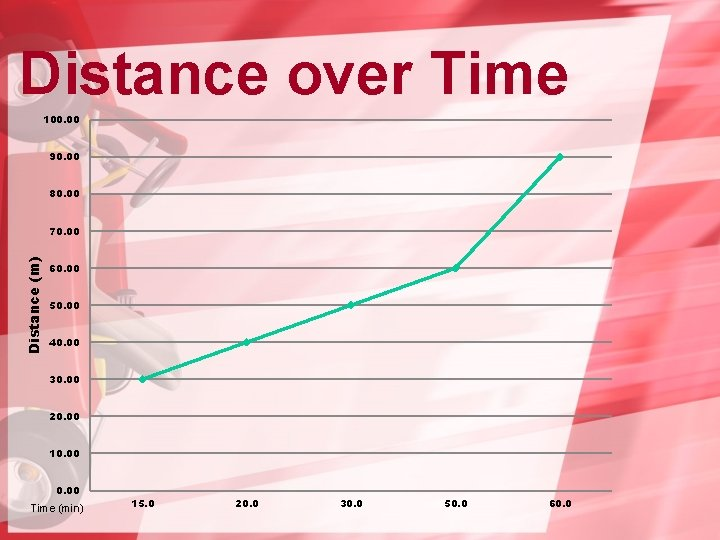Distance over Time 100. 00 90. 00 80. 00 Distance (m) 70. 00 60. 00 50. 00 40. 00 30. 00 20. 00 10. 00 Time (min) 15. 0 20. 0 30. 0 50. 0 60. 0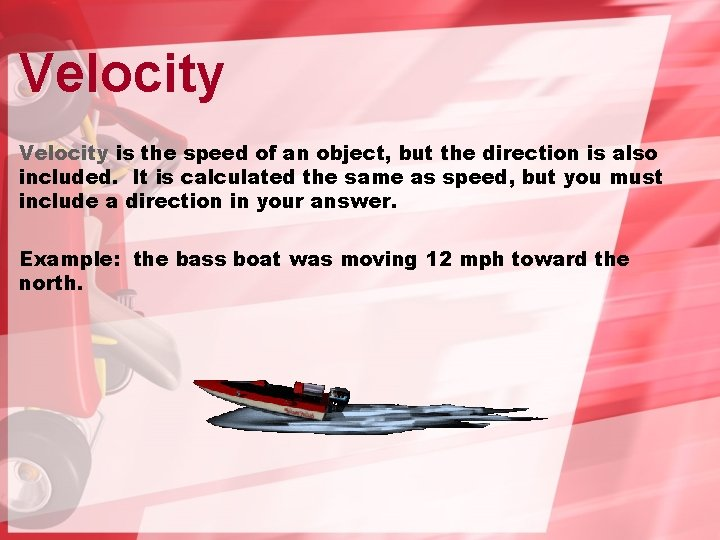Velocity is the speed of an object, but the direction is also included. It is calculated the same as speed, but you must include a direction in your answer. Example: the bass boat was moving 12 mph toward the north.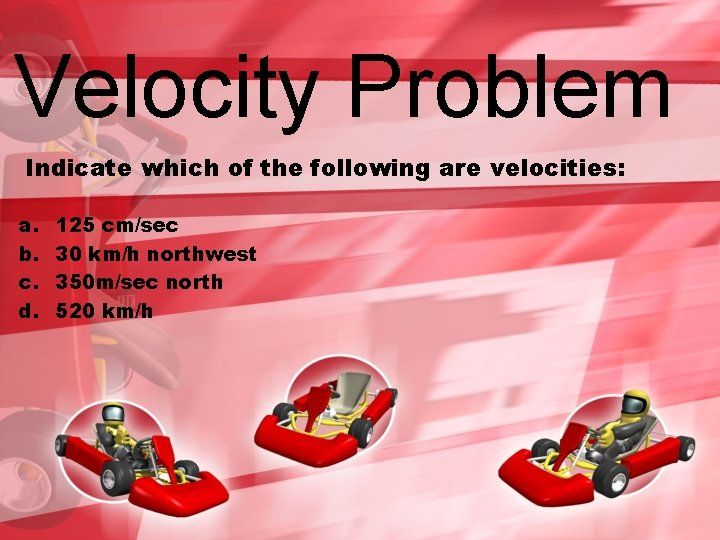Velocity Problem Indicate which of the following are velocities: a. b. c. d. 125 cm/sec 30 km/h northwest 350 m/sec north 520 km/h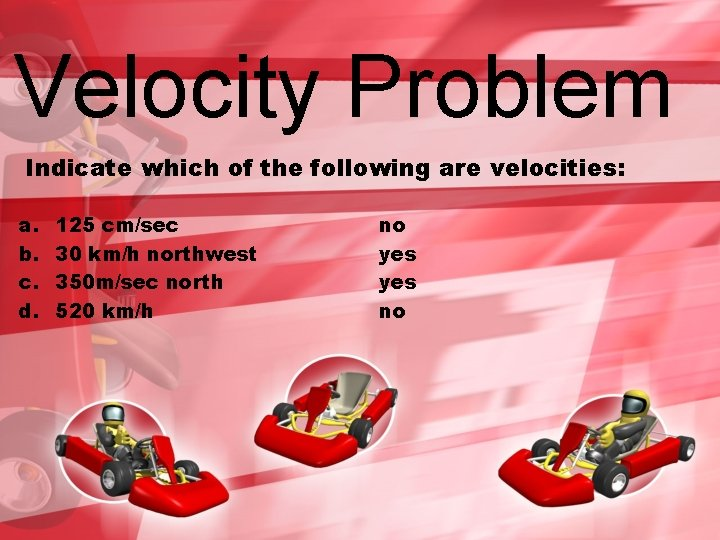Velocity Problem Indicate which of the following are velocities: a. b. c. d. 125 cm/sec 30 km/h northwest 350 m/sec north 520 km/h no yes no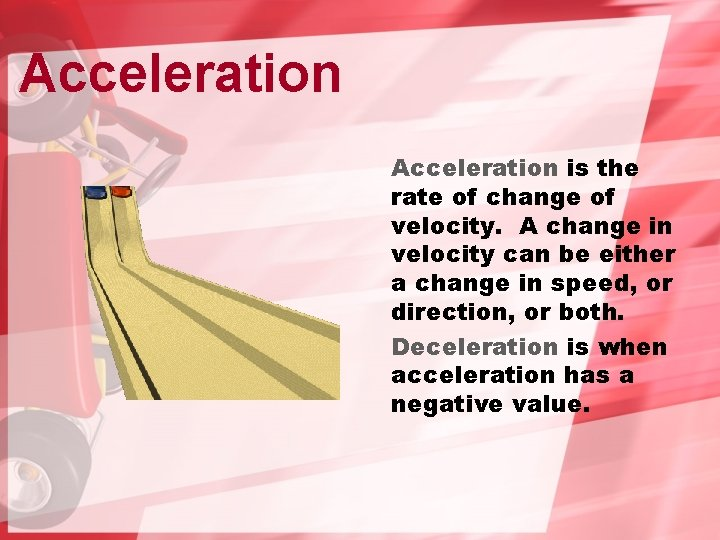Acceleration is the rate of change of velocity. A change in velocity can be either a change in speed, or direction, or both. Deceleration is when acceleration has a negative value.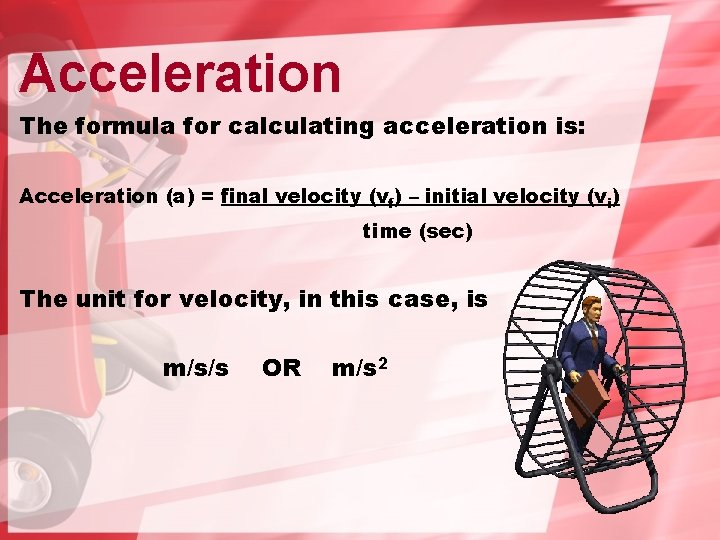Acceleration The formula for calculating acceleration is: Acceleration (a) = final velocity (vf) – initial velocity (vi) time (sec) The unit for velocity, in this case, is m/s/s OR m/s 2Acceleration Math Problem A jet starts at rest at the end of a runway and reaches a speed of 80 m/s in 20 s. What is its acceleration?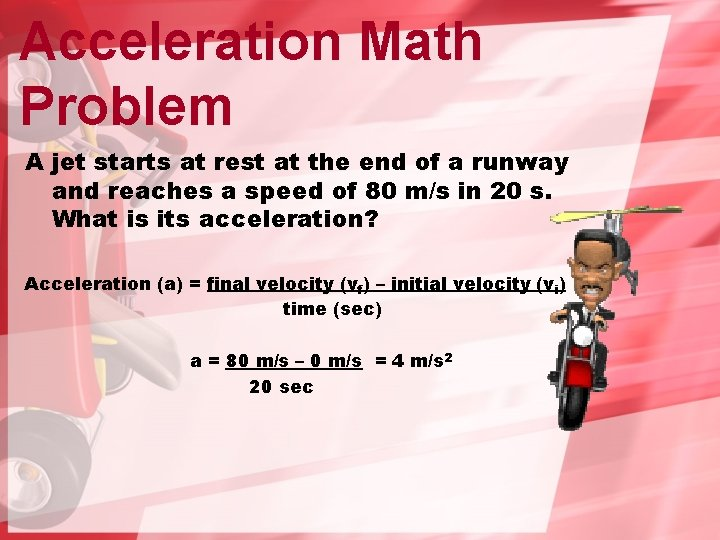Acceleration Math Problem A jet starts at rest at the end of a runway and reaches a speed of 80 m/s in 20 s. What is its acceleration? Acceleration (a) = final velocity (vf) – initial velocity (vi) time (sec) a = 80 m/s – 0 m/s = 4 m/s 2 20 sec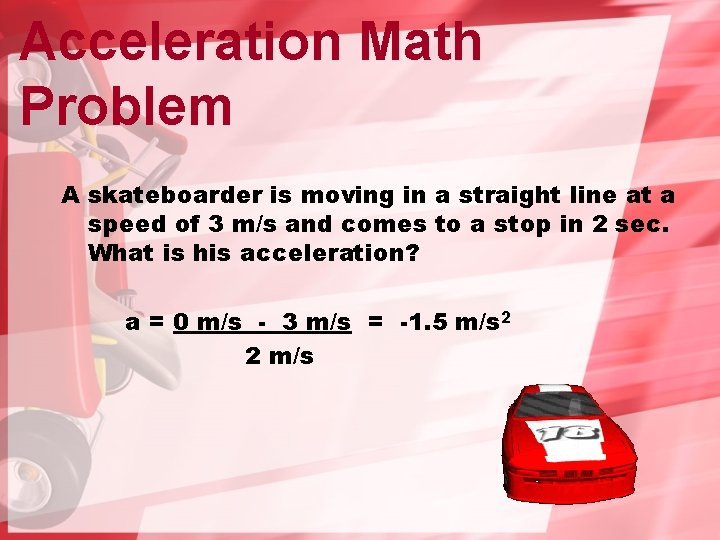Acceleration Math Problem A skateboarder is moving in a straight line at a speed of 3 m/s and comes to a stop in 2 sec. What is his acceleration? a = 0 m/s - 3 m/s = -1. 5 m/s 2 2 m/sHomework Assignment Handout on calculating speed and velocity Handout on average speed Handout on acceleration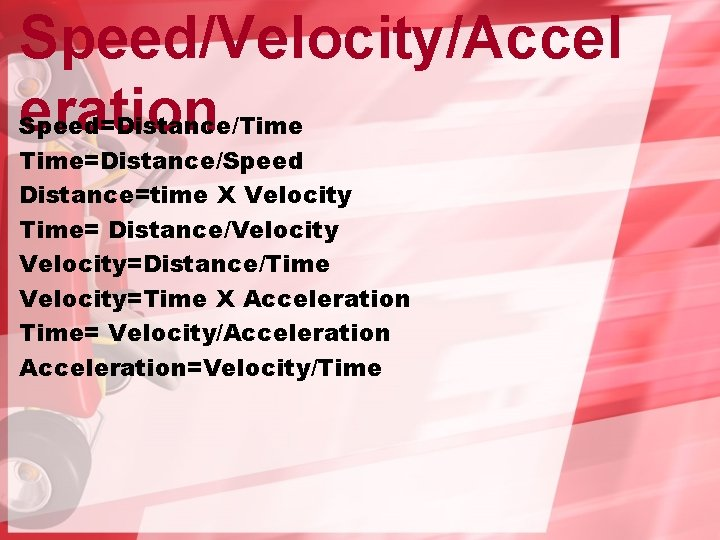Speed/Velocity/Accel eration Speed=Distance/Time=Distance/Speed Distance=time X Velocity Time= Distance/Velocity=Distance/Time Velocity=Time X Acceleration Time= Velocity/Acceleration=Velocity/TimeThe End!